## Icy wall effect

This section shows you how to implement the icy wall effect in your own Unity programs.

The Ice Cave demo uses a reflection effect in the icy walls of the cave. This effect is subtle but adds extra realism and atmosphere to the scene.

Ice can be a difficult material to replicate because light scatters off it in different ways, depending on the small details of its surface. The reflection can be completely clear, completely distorted, or anywhere in between. The Ice Cave demo shows this effect and includes a parallax effect for greater realism. The following image shows the Ice Cave demo, demonstrating the icy wall effect: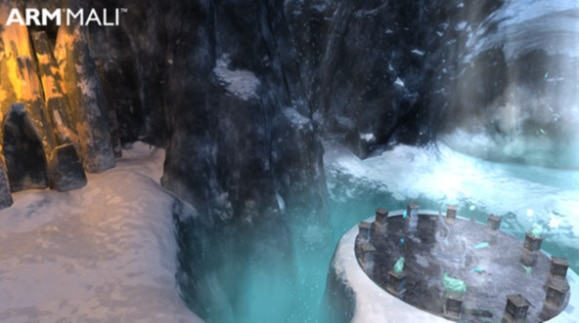The following image shows a close up of this effect: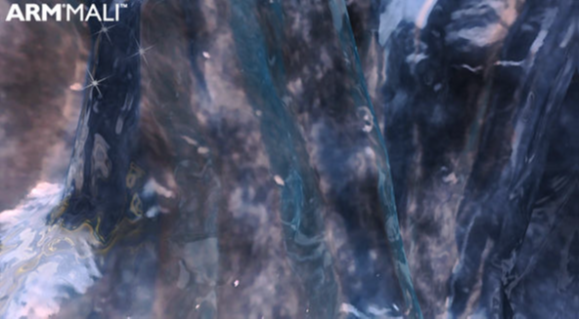#### Modifying and combining normal maps to affect reflections

The reflection effect in the Ice Cave demo uses the tangent space normal maps and calculated gray scale fake normal maps. Combining both maps with some modifiers creates the effect in the demo.

The gray scale fake normal maps are a gray scale of the tangent space normal maps. In the Ice Cave demo, most values in the gray scale maps are in the range 0.3-0.8. The following image shows the tangent space normal maps and gray scale fake normal maps that the Ice Cave demo uses: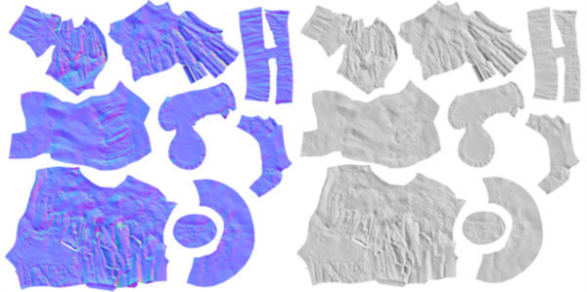#### Why tangent space normal maps are used?

The tangent space normal maps are used in the Ice Cave demo, because they have a small range of values in the resulting gray scale. Therefore, the tangent space normal maps work well in the later stages of this rendering process.

An alternative to tangent space normal maps is to use object space normal maps. These maps show the same details that the tangent space normal maps show, but also show where light hits. Therefore, the range of values in the resulting object space normal map gray scale is too large to work well in the later stages of the rendering process. The following image shows this effect: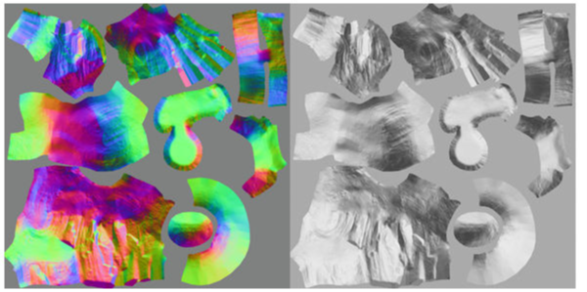#### Applying transparency to parts of the normal maps

The gray scale fake normal maps are only applied to the areas without snow. To prevent them from being applied to the snowy areas, the gray scale fake normal maps are modified using the diffuse texture maps for the same surfaces. This modification increases the alpha component of the gray scale fake normal maps where snow appears in the texture maps.

The following image shows the diffuse texture maps for the Ice Cave demo static surfaces and the result when they are applied to the gray scale fake normal maps: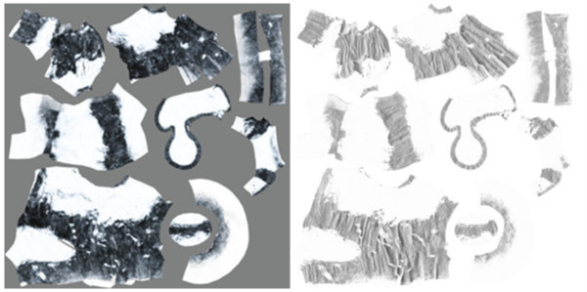#### Creating the reflections from the different normal maps

The combination of the transparency-adjusted gray scale fake normal maps and the true normal maps creates the reflection effects that are shown in the Ice Cave demo.

The transparency-adjusted gray scale fake normal maps, `bumpFake`, and the true normal maps, `bumpNorm`, are combined proportionally. The combination uses the following function:

`half4 bumpNormalFake = lerp(bumpNorm, bumpFake, amountFakeNormalMap);`

This code means that in the darker parts of the cave, the main reflection contribution comes from the gray scale fake normals. In the snowy parts of the cave, the effect comes from the object space normals. To apply the effect using the gray scale fake normal maps, the gray scale must be converted into normal vectors. To start this process, the three components of the normal vectors are set equal to the gray scale value. In the Ice Cave demo, the components vectors are between (0.3, 0.3, 0.3) and (0.8, 0.8, 0.8). This is because all the components are set so that they are the same and all the normal vectors point in the same direction.

The shader applies a transformation to normal components. This uses the transform that is usually used to transform values in the range 0-1 to the range -1 to 1. The equation which performs this change is the following:

`result = 2 * value - 1. `

This equation changes the normal vectors so that they either point in the direction they pointed before, or the opposite direction. For example, if the original has the components (0.3, 0.3, 0.3), this means that the resulting normal is (-0.4, -0.4, -0.4). If the original has the components (0.8, 0.8, 0.8), this means that the resulting normal is (0.6, 0.6, 0.6).

After the transformation to the range -1 to 1, the vectors are fed to the `reflect()` function. This function is designed to work using normalized normal vectors. However, in this case the non-normalized normal is passed to the function. The following code shows how the shader built-in function `reflect()` works:

`R = reflect(I, N) = I – 2 * dot(I, N) * N`

Using this function with a non-normalized input normal with a length less than one, causes the reflection vector to deviate more than expected from the normal. This is because of what the reflection law states.

When the value of the components of the normal vector goes below 0.5, the reflection vector switches to the opposite direction. When the switch takes place the reflection vector switches to the opposite direction, a different part of the cubemap is read. This switching between different parts of the cubemap creates the effect of uneven spots. The uneven spots reflect the white parts of the cubemap next to the reflections of the rocky parts of the cubemap. This is because the areas in the gray scale fake normal maps that cause the switches between positive and negative normal are also the areas that produce the most distorted angles on the reflected vectors. This creates an appealing swirl effect. The following image shows this effect: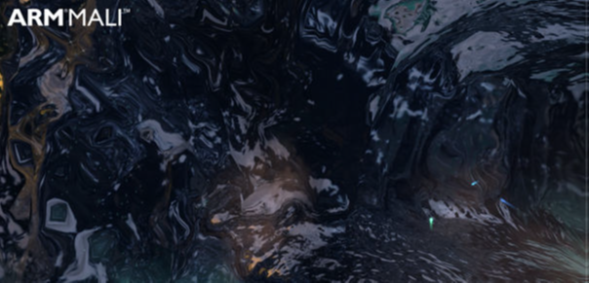If the shader is programmed to use the fake normal values without the non-normalized clamped stage, the result features diagonal bands. The result featuring diagonal bands is a significant difference and shows that these stages are important. The following image shows the resulting effect without the clamp stage: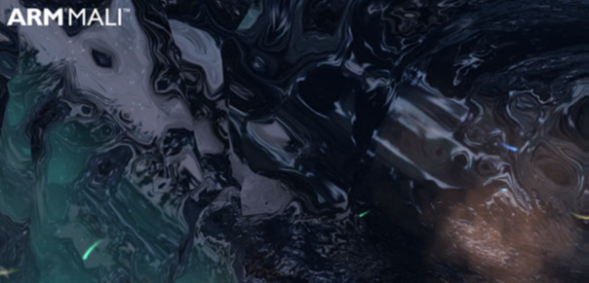#### Applying local correction to the reflections

Applying local correction to the reflection vector improves the realism of the effect. Without this correction, the reflection does not change with the position of the camera like reflections in real life do. This is especially true for lateral movements of the camera. For more information, see Generating correct reflections with a local cubemap.